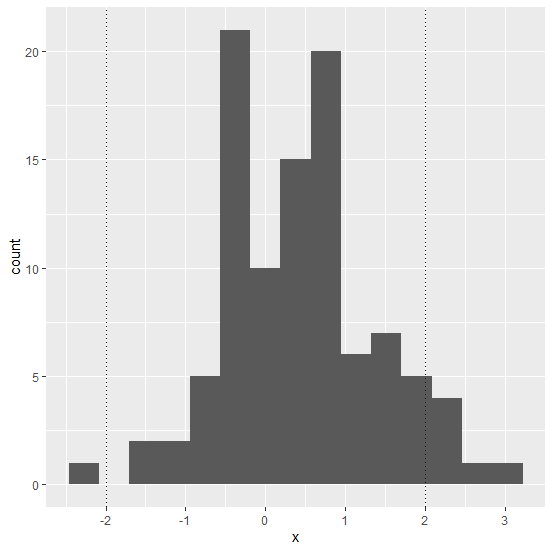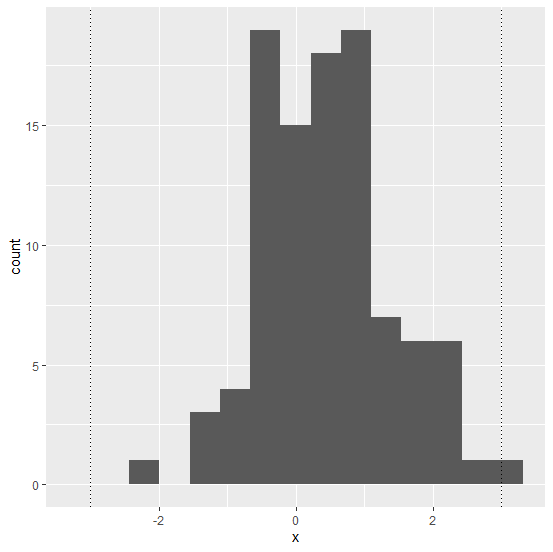# How to create dotted vertical lines in a plot using ggplot2 in R?

In any plot, the vertical lines are generally used to show the thresholds for something, for example, range of the variable under consideration. The package ggplot2 provides geom_vline function to create vertical lines on a plot and we have linetype argument of this function which can be used to draw dotted vertical lines.

## Example

Consider the below data frame −

set.seed(9)
x <-rnorm(100,0.5)
df <-data.frame(x)

Creating histogram of x with dotted vertical lines −

## Example

library(ggplot2)
ggplot(df,aes(x))+geom_histogram(bins=15)+geom_vline(xintercept=c(-2,2),linetype="dotted")

## Output## Example

ggplot(df,aes(x))+geom_histogram(bins=15)+geom_vline(xintercept=c(-3,3),linetype="dotted")

## Output